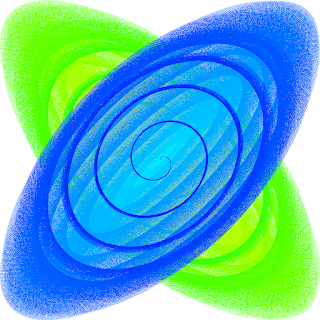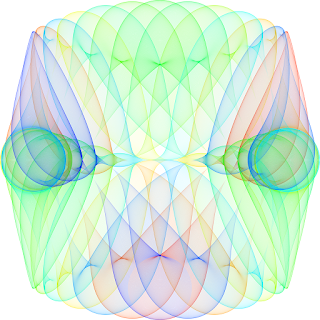## Sunday, 20 September 2015

### Math Art Work with just segments and circles

This post was inspired by the article in the CNN in the following link.

Using a big number of segments or circles we can create nice art work like the images below. When a number of segments under a certain parametrization intersect at some points, the set of intersection points define a curve on the plane called the evolute.

For example, imagine a fixed length bar which stands vertical on the Y-axis. Then, we take the extreme of the bar at (0,0) and move it through the X-axis, while letting the other extreme descend through the Y-axis. The intersection of all this segment will define a curve, and the segments are tangent to the curve at each of the intersection points. Many curves such as parabola, hyperbola can be constructed in this way.python mathart.py -c black -C black -n 100 -o "artwork10.png" -e line -X1 "0.0" -Y1 "sin(pi/2.0*k/n)*(-1.0)" -X2 "cos(pi/2.0*k/n)" -Y2 "0.0"

Using more complex parametrizations as the one described in the origina article and in the script.sh file, and letting our imagination fly, we can come up with nice geometrical figures.

When I first tackled this problem with the very first lines of code at the early stage of the program, I observed that the images I obtained where fuzzy. If I increased the number of elements to get more defined curves, what I got was large areas of pixels of the same color.  This is due to the fact that computers image are a set of discrete points instead of a continuum of points. When drawing many elements the non-integer coordenates will be plotted at the nearest pixel, creating an overlapping, as we can appreciate on the picture below. This effect is known in signal processing as the Aliasing problem.Example of figure without using anti-aliasing technique.

The solution to this problem is obviously to increase the resolution of the image.  In order to increase the resolution while preserving the same image size, what we do is to create a first image larger than our final image. All calculations are done in this larger image, larger by a factor specified on the command line of the program as super-sampling. Once we have this larger image, we resize or down-size the image to the size we originally wanted. In order to down-size the image, we can choose amongst several algorithms to apply: Nearest, Bilinear, Bicubic, Antialias. In this case we use the Antialias filtering which is the one resulting in higher quality images in this case.

Using this super-sampling technique, we come up with much better quality pictures. The pictures below have been created with the Python script at the end of this post. The legend of each pictures include the command-line used to create the picture. All these examples are under the script.sh file on the same directory.

You can get the code from my github repository at the following link.python mathart.py -c red -C blue -n 25000 -o "artwork1.png" -e line -X1 "sin(108.0*pi*k/n)*sin(4.0*pi*k/n)" -Y1 "cos(106.0*pi*k/n)*sin(4.0*pi*k/n)" -X2 "sin(104.0*pi*k/n)*sin(4.0*pi*k/n)" -Y2 "cos(102.0*pi*k/n)*sin(4.0*pi*k/n)"python mathart.py -c black -C blue -n 25000 -o "artwork2.png" -e line -X1 "3.0/4.0*cos(86.0*pi*k/n)" -Y1 "sin(84.0*pi*k/n)**5" -X2 "sin(82.0*pi*k/n)**5" -Y2 "3.0/4.0*cos(80.0*pi*k/n)"python mathart.py -c red -C blue -n 25000 -o "artwork3.png" -e circle -Xc "sin(14.0*pi*k/n)" -Yc "cos(26.0*pi*k/n)**3" -R "1.0/4.0*cos(40.0*pi*k/n)**2"python mathart.py -c red -C blue -n 25000 -o "artwork4.png" -e circle -Xc "cos(6.0*pi*k/n)" -Yc "sin(20.0*pi*k/n)**3" -R "1.0/4.0*cos(42.0*pi*k/n)**2"python mathart.py -c red -C blue -n 25000 -o "artwork5.png" -e circle -Xc "cos(6.0*pi*k/n)" -Yc "sin(14.0*pi*k/n)**3" -R "1.0/4.0*cos(66.0*pi*k/n)**2"python mathart.py -c black -C blue -n 25000 -o "artwork6.png" -e circle -Xc "cos(14.0*pi*k/n)**3" -Yc "sin(24.0*pi*k/n)**3" -R "1.0/3.0*cos(44.0*pi*k/n)**4"python mathart.py -c red -C green -n 25000 -o "artwork7.png" -e circle -Xc "sin(22.0*pi*k/n)**3" -Yc "cos(6.0*pi*k/n)" -R "1.0/5.0*cos(58.0*pi*k/n)**2"python mathart.py -c yellow -C blue -n 25000 -o "artwork8.png" -e circle -Xc "cos(2.0*pi*k/n)" -Yc "sin(18.0*pi*k/n)**3" -R "1.0/4.0*cos(42.0*pi*k/n)**2"

The Python code is include below.

## Wednesday, 16 September 2015

### Diffusion Limited Aggregation and Brownian Trees

Diffusion limited aggregation (DLA) is a type of stochastic process in which particles move following a Brownian motion until they stick together with other particles.

Imagine a square in which we have put some glue on the bottom edge. Then, we release one particle at a time on that square. The particles follow a random-walk within the square until they stick to the bottom edge or to a particle already fixed or rooted. Then, that particle becomes itself attached to the fixed structure. This fixed structure so formed is commonly called Brownian trees, because it recalls the shape of a tree.

Many natural phenomena, such as electrodeposition, dielectric breakdown (lightings), coral growth, snowflake formation and other not so natural such as urban areas growth can be simulated using DLA. To the basic diffusion process, we can add other physical features such as gravitation, external forces, attraction between particles, fluids, different frames where particles can be attached... to achieve different results.

The snippet of code below is an example of a simple two dimensional DLA simulation written in Python.

The following images were created using the script above, for different type of frames.

On the bit-player.org blog, we can find interesting examples of such processes. There are even several programming libraries to simulate such processes. As usual, adding a litte bit of imagination we can easily hack the script above to generate new wonderful images.

## Thursday, 10 September 2015

### Benford Law in Stock Market Index

The Benford's Law is an empirical law about the probability distribution of the first digit of each number. In everyday datasets, especially ones with values with different order of magnitude,  such as bill, number in addresses, populations, stock market prices and so on, the probability of the first digit is not uniform as one could think. Instead, the probability of the first digit d is proportional to the space between d and d+1 in logarithmic space. Thus, the probability of the digit d, being d a digit in [1,9] is a decreasing function which can be calcualted as:

For a more detailed description of the Law, I strongly recommend to visit the wikipedia entry for the Benford's Law.

Being a little curious about this law, I wrote a short R script to check this law against the prices of stocks in the different stock market indexes, such as S&P 500, DJ EUROSTOXX50E, FTSE 100, IBEX 35, RUSSELL 2000 and NIKKEI 225. In order to run this script, you will need a computer with a Bloomberg connection. I guess it can be easily hacked so that we can get the stock prices from the Yahoo! finance website, but I haven't tried.

library(Rbbg) #Bloomberg Connection

#Connect to Bloomberg API
conn <- blpConnect()

indexes <- c("RTY Index", "SX5E Index", "IBEX Index", "SPX Index", "NKY Index", "UKX Index")

#Get members for index and their RICS
memb_codes <- bds(conn, indexes, c("INDX_MEMBERS") )
rics <- paste(memb_codes[ ,1], "Equity", sep= " ")

#Get spots for each member and their first digit
spots <- bdp(conn, rics, c("PX_LAST") )
firstDigit <- as.numeric( substring( spots[ , 1], 1, 1 ) )

#Get histogram for first Digit
histogram <- hist( firstDigit, breaks = 0:9 )

#Benford Law Theoretical Probability
benfordTheoretical <- log10( 1 + 1/(1:9) )

#Result dataframe
results_UKX <- data.frame(FirstDigit = 1:9, Probability = histogram$density, Theoretical = benfordTheoretical) #For those not having a bloomberg connection, we can load the data load("BenfordLaw.RData") #Plot results plot(results_UKX$Probability, main='Benford Law for stock prices in FTSE100 Index',
xlab="First Digit", ylab="Probability", col="green", pch=1, xaxt="n")
axis(1, at=seq(1,9))
lines(results_UKX\$Theoretical, col="red", pch=0)
legend("topright", c("Results", "Theoretical"), lty=c(0,1), pch=c(1,0), col=c("green", "red"))

I attach the results for each of the index to see how well the distribution matches the theoretical Benford distribution.

## Tuesday, 8 September 2015

### GPX Reading for latter processing

Whenever I go for hiking, running or climbing to the mountains, I usually record all my tracks with a GPS, so, I end up with hundred of GPS files on my computer. GPX is the universal format to store GPS track data, and it has been defined as an schema of XML. The definition of the GPX format is described in the topographix website.

In order to extract interesting data from all the tracks I have along these years, I have written several snippets of code in different languages to convert the GPX file to another format better adapted for further processing. Once we have the track data in a suitable format, we can look deeper into the data of our outdoor activities, and even apply some machine learning on them to get interesting information, as we will see in future posts.

When dealing with GPX files, first option should always be to have a look at the great open-source GPSBabel. GPSBabel is a command line application able to translate GPS data between a plethora of formats. GPSBabel is usually launched directly from a terminal windows, but, as a first step to deal with  GPX data, we can also call it from another language such as R or Python.

In the case of R language, we can use the system() call function to launch the gpsbabel command with the correct arguments so that we read a .gpx file and convert it to a text file. We can apply the filters provided by gpsbabel to include or exclude waypoints, routes or other information within the gpx we are not interested in. The system() call will return a file (pipe) descriptor which can be read with the textConnection data. Then, passing the return of textConnection() to the read.table() function, we will have a data.frame object with the points of the GPS track. Further processing will be needed to extract the columns we are interested in.

A better and simpler option is to read directly the GPX file from R, using the XML library. The XML library allows to parse XML file and run X-Path query on the data. With four simple X-Path query, we will be able to read the latitude, longitude, elevation and timestamp information for each of the points in the GPS track. If there is more information on the file, for example, Suunto watches also stores heart bpm, we can easily modify the code so that we include this information as well.  The following code shows how to apply this method in R. The code is part of a bigger project in R which I will release as an R-package:

Sometimes I have needed to do some processing directly from the command line. To that purpose, I wrote this bash script using xmllint and sed which extracts the information from the GPX file and store it in a text output file.

Using an XML library able to query X-Path expressions, is easy to extend this method to other languages such as Python or C++. I will add the code at some time in the future at this post If I ever need to write such functions in other programming languages.